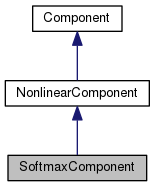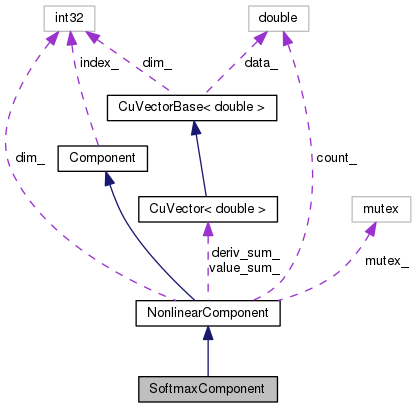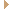SoftmaxComponent Class Reference

`#include <nnet-component.h>`

Inheritance diagram for SoftmaxComponent:[legend]
Collaboration diagram for SoftmaxComponent:[legend]

## Public Member Functions

SoftmaxComponent (int32 dim)

SoftmaxComponent (const SoftmaxComponent &other)

SoftmaxComponent ()

virtual std::string Type () const

virtual bool BackpropNeedsInput () const

virtual bool BackpropNeedsOutput () const

virtual void Propagate (const ChunkInfo &in_info, const ChunkInfo &out_info, const CuMatrixBase< BaseFloat > &in, CuMatrixBase< BaseFloat > *out) const
Perform forward pass propagation Input->Output. More...

virtual void Backprop (const ChunkInfo &in_info, const ChunkInfo &out_info, const CuMatrixBase< BaseFloat > &in_value, const CuMatrixBase< BaseFloat > &out_value, const CuMatrixBase< BaseFloat > &out_deriv, Component *to_update, CuMatrix< BaseFloat > *in_deriv) const
Perform backward pass propagation of the derivative, and also either update the model (if to_update == this) or update another model or compute the model derivative (otherwise). More...

void MixUp (int32 num_mixtures, BaseFloat power, BaseFloat min_count, BaseFloat perturb_stddev, AffineComponent *ac, SumGroupComponent *sc)
Allocate mixtures to states via a power rule, and add any new mixtures. More...

virtual ComponentCopy () const
Copy component (deep copy). More...Public Member Functions inherited from NonlinearComponent
void Init (int32 dim)

NonlinearComponent (int32 dim)

NonlinearComponent ()

NonlinearComponent (const NonlinearComponent &other)

virtual int32 InputDim () const
Get size of input vectors. More...

virtual int32 OutputDim () const
Get size of output vectors. More...

virtual void InitFromString (std::string args)
We implement InitFromString at this level. More...

virtual void Read (std::istream &is, bool binary)
We implement Read at this level as it just needs the Type(). More...

virtual void Write (std::ostream &os, bool binary) const
Write component to stream. More...

void Scale (BaseFloat scale)

void Add (BaseFloat alpha, const NonlinearComponent &other)

const CuVector< double > & ValueSum () const

const CuVector< double > & DerivSum () const

double Count () const

void SetDim (int32 dim)Public Member Functions inherited from Component
Component ()

virtual int32 Index () const
Returns the index in the sequence of layers in the neural net; intended only to be used in debugging information. More...

virtual void SetIndex (int32 index)

virtual std::vector< int32Context () const
Return a vector describing the temporal context this component requires for each frame of output, as a sorted list. More...

void Propagate (const ChunkInfo &in_info, const ChunkInfo &out_info, const CuMatrixBase< BaseFloat > &in, CuMatrix< BaseFloat > *out) const
A non-virtual propagate function that first resizes output if necessary. More...

virtual std::string Info () const

virtual ~Component ()

## Private Member Functions

SoftmaxComponentoperator= (const SoftmaxComponent &other)Static Public Member Functions inherited from Component
static ComponentReadNew (std::istream &is, bool binary)

static ComponentNewFromString (const std::string &initializer_line)
Initialize the Component from one line that will contain first the type, e.g. More...

static ComponentNewComponentOfType (const std::string &type)
Return a new Component of the given type e.g. More...Protected Member Functions inherited from NonlinearComponent
void UpdateStats (const CuMatrixBase< BaseFloat > &out_value, const CuMatrixBase< BaseFloat > *deriv=NULL)

const NonlinearComponentoperator= (const NonlinearComponent &other)Protected Attributes inherited from NonlinearComponent
int32 dim_

CuVector< double > value_sum_

CuVector< double > deriv_sum_

double count_

std::mutex mutex_

## Detailed Description

Definition at line 777 of file nnet-component.h.

## ◆ SoftmaxComponent() [1/3]

 SoftmaxComponent ( int32 dim )
inlineexplicit

Definition at line 779 of file nnet-component.h.

## ◆ SoftmaxComponent() [2/3]

 SoftmaxComponent ( const SoftmaxComponent & other )
inlineexplicit

Definition at line 780 of file nnet-component.h.

## ◆ SoftmaxComponent() [3/3]

 SoftmaxComponent ( )
inline

Definition at line 781 of file nnet-component.h.

781 { }

## ◆ Backprop()

 void Backprop ( const ChunkInfo & in_info, const ChunkInfo & out_info, const CuMatrixBase< BaseFloat > & in_value, const CuMatrixBase< BaseFloat > & out_value, const CuMatrixBase< BaseFloat > & out_deriv, Component * to_update, CuMatrix< BaseFloat > * in_deriv ) const
virtual

Perform backward pass propagation of the derivative, and also either update the model (if to_update == this) or update another model or compute the model derivative (otherwise).

Note: in_value and out_value are the values of the input and output of the component, and these may be dummy variables if respectively BackpropNeedsInput() or BackpropNeedsOutput() return false for that component (not all components need these).

num_chunks lets us treat the input matrix as contiguous-in-time chunks of equal size; it only matters if splicing is involved.

Implements Component.

Definition at line 919 of file nnet-component.cc.

925  {
926  /*
927  Note on the derivative of the softmax function: let it be
928  p_i = exp(x_i) / sum_i exp_i
929  The [matrix-valued] Jacobian of this function is
930  diag(p) - p p^T
931  Let the derivative vector at the output be e, and at the input be
932  d. We have
933  d = diag(p) e - p (p^T e).
934  d_i = p_i e_i - p_i (p^T e).
935  */
936  in_deriv->Resize(out_deriv.NumRows(), out_deriv.NumCols());
937  in_deriv->DiffSoftmaxPerRow(out_value, out_deriv);
938
939  // The SoftmaxComponent does not have any real trainable parameters, but
940  // during the backprop we store some statistics on the average counts;
941  // these may be used in mixing-up.
942  if (to_update != NULL) {
943  NonlinearComponent *to_update_nonlinear =
944  dynamic_cast<NonlinearComponent*>(to_update);
946  }
947 }

## ◆ BackpropNeedsInput()

 virtual bool BackpropNeedsInput ( ) const
inlinevirtual

Reimplemented from Component.

Definition at line 783 of file nnet-component.h.

783 { return false; }

## ◆ BackpropNeedsOutput()

 virtual bool BackpropNeedsOutput ( ) const
inlinevirtual

Reimplemented from Component.

Definition at line 784 of file nnet-component.h.

References Component::Propagate().

784 { return true; }

## ◆ Copy()

 virtual Component* Copy ( ) const
inlinevirtual

Copy component (deep copy).

Implements Component.

Definition at line 805 of file nnet-component.h.

805 { return new SoftmaxComponent(*this); }

## ◆ MixUp()

 void MixUp ( int32 num_mixtures, BaseFloat power, BaseFloat min_count, BaseFloat perturb_stddev, AffineComponent * ac, SumGroupComponent * sc )

Allocate mixtures to states via a power rule, and add any new mixtures.

Total the count out of

all the output dims of the softmax layer that correspond to this mixture. We'll use this total to allocate new quasi-Gaussians.

Definition at line 107 of file mixup-nnet.cc.

Referenced by kaldi::nnet2::MixupNnet().

112  {
113  // "counts" is derived from this->counts_ by summing.
114  std::vector<int32> old_sizes;
115  sc->GetSizes(&old_sizes);
116  Vector<BaseFloat> counts(old_sizes.size());
117  int32 old_dim = 0;
118  for (size_t i = 0; i < old_sizes.size(); i++) {
119  int32 this_input_dim = old_sizes[i];
120  BaseFloat this_tot_count = 0.0;
121  for (int32 d = 0; d < this_input_dim; d++, old_dim++)
124  this_tot_count += this->value_sum_(old_dim);
125  counts(i) = this_tot_count;
126  }
127  KALDI_ASSERT(old_dim == value_sum_.Dim());
128  KALDI_ASSERT(counts.Sum() > 0 && "Cannot do mixing up without counts.");
129
130  std::vector<int32> targets; // #mixtures for each state.
131
132
133  // Get the target number of mixtures for each state.
134  GetSplitTargets(counts, num_mixtures, power, min_count, &targets);
135  KALDI_ASSERT(targets.size() == old_sizes.size());
136  std::vector<int32> new_sizes(old_sizes.size());
137  for (size_t i = 0; i < targets.size(); i++)
138  new_sizes[i] = std::max(targets[i], old_sizes[i]);
139  int32 new_dim = std::accumulate(new_sizes.begin(), new_sizes.end(),
140  static_cast<int32>(0)),
141  affine_input_dim = ac->InputDim();
142  KALDI_ASSERT(new_dim >= old_dim);
143  sc->Init(new_sizes);
144
145  // bias and linear terms from affine component:
146  Vector<BaseFloat> old_bias_term(ac->bias_params_);
147  Matrix<BaseFloat> old_linear_term(ac->linear_params_);
148
149  Vector<BaseFloat> new_bias_term(new_dim);
150  Matrix<BaseFloat> new_linear_term(new_dim, affine_input_dim);
151  Vector<BaseFloat> new_counts(new_dim);
152
153  // old_offset and new_offset are offsets into the dimension at the
154  // input/output of the softmax component, before and after mixing up
155  // respectively. They get incremented in the following loop.
156  int32 old_offset = 0, new_offset = 0;
157  Vector<BaseFloat> old_counts(this->value_sum_);
158  for (size_t i = 0; i < old_sizes.size(); i++) {
159  int32 this_old_dim = old_sizes[i],
160  this_new_dim = new_sizes[i],
161  this_cur_dim = this_old_dim; // this_cur_dim is loop variable.
162
163  SubMatrix<BaseFloat> this_old_linear_term(old_linear_term,
164  old_offset, this_old_dim,
165  0, affine_input_dim),
166  this_new_linear_term(new_linear_term,
167  new_offset, this_new_dim,
168  0, affine_input_dim);
169  SubVector<BaseFloat> this_old_bias_term(old_bias_term,
170  old_offset, this_old_dim),
171  this_new_bias_term(new_bias_term, new_offset, this_new_dim),
172  this_old_counts(old_counts,
173  old_offset, this_old_dim),
174  this_new_counts(new_counts,
175  new_offset, this_new_dim);
176
177  // Copy the same-dimensional part of the parameters and counts.
178  this_new_linear_term.Range(0, this_old_dim, 0, affine_input_dim).
179  CopyFromMat(this_old_linear_term);
180  this_new_bias_term.Range(0, this_old_dim).
181  CopyFromVec(this_old_bias_term);
182  this_new_counts.Range(0, this_old_dim).
183  CopyFromVec(this_old_counts);
184  // this_new_params is the mixture weights.
185  // Add the new components...
186  for (; this_cur_dim < this_new_dim; this_cur_dim++) {
187  BaseFloat *count_begin = this_new_counts.Data(),
188  *count_end = count_begin + this_cur_dim,
189  *count_max = std::max_element(count_begin, count_end);
190  KALDI_ASSERT(*count_max > 0.0);
191  *count_max *= 0.5;
192  *count_end = *count_max; // count for the element we're adding.
193  int32 max_index = static_cast<int32>(count_max - count_begin),
194  new_index = this_cur_dim;
195  SubVector<BaseFloat> cur_vec(this_new_linear_term, max_index),
196  new_vec(this_new_linear_term, new_index);
197  new_vec.CopyFromVec(cur_vec);
198  Vector<BaseFloat> rand(affine_input_dim);
199  rand.SetRandn();
202  this_new_bias_term(max_index) += Log(0.5);
203  this_new_bias_term(new_index) = this_new_bias_term(max_index);
204  }
205  old_offset += this_old_dim;
206  new_offset += this_new_dim;
207  }
208  KALDI_ASSERT(old_offset == old_dim && new_offset == new_dim);
209  ac->SetParams(new_bias_term, new_linear_term);
210  this->value_sum_.Resize(new_counts.Dim());
211  this->value_sum_.CopyFromVec(new_counts);
212  this->count_ = this->value_sum_.Sum();
213  this->dim_ = new_dim;
214  KALDI_LOG << "Mixed up from dimension of " << old_dim << " to " << new_dim
215  << " in the softmax layer.";
216 }
Real Sum() const
Definition: cu-vector.cc:297
kaldi::int32 int32
void GetSplitTargets(const Vector< BaseFloat > &state_occs, int32 target_components, BaseFloat power, BaseFloat min_count, std::vector< int32 > *targets)
Get Gaussian-mixture or substate-mixture splitting targets, according to a power rule (e...
float BaseFloat
Definition: kaldi-types.h:29
void CopyFromVec(const CuVectorBase< Real > &src)
Copy functions; these will crash if the dimension do not match.
Definition: cu-vector.cc:1078
double Log(double x)
Definition: kaldi-math.h:100
void Resize(MatrixIndexT dim, MatrixResizeType t=kSetZero)
Allocate the memory.
Definition: cu-vector.cc:993
#define KALDI_ASSERT(cond)
Definition: kaldi-error.h:185
#define KALDI_LOG
Definition: kaldi-error.h:153
MatrixIndexT Dim() const
Dimensions.
Definition: cu-vector.h:69

## ◆ operator=()

 SoftmaxComponent& operator= ( const SoftmaxComponent & other )
private

## ◆ Propagate()

 void Propagate ( const ChunkInfo & in_info, const ChunkInfo & out_info, const CuMatrixBase< BaseFloat > & in, CuMatrixBase< BaseFloat > * out ) const
virtual

Perform forward pass propagation Input->Output.

Each row is one frame or training example. Interpreted as "num_chunks" equally sized chunks of frames; this only matters for layers that do things like context splicing. Typically this variable will either be 1 (when we're processing a single contiguous chunk of data) or will be the same as in.NumFrames(), but other values are possible if some layers do splicing.

Implements Component.

Definition at line 900 of file nnet-component.cc.

903  {
904  in_info.CheckSize(in);
905  out_info.CheckSize(*out);
906  KALDI_ASSERT(in_info.NumChunks() == out_info.NumChunks());
907
908  // Apply softmax function to each row of the output...
909  // for that row, we do
910  // x_i = exp(x_i) / sum_j exp(x_j).
911
912  out->SoftMaxPerRow(in);
913
914  // This floor on the output helps us deal with
915  // almost-zeros in a way that doesn't lead to overflow.
916  out->ApplyFloor(1.0e-20);
917 }
#define KALDI_ASSERT(cond)
Definition: kaldi-error.h:185

## ◆ Type()

 virtual std::string Type ( ) const
inlinevirtual

Implements Component.

Definition at line 782 of file nnet-component.h.

782 { return "SoftmaxComponent"; }

The documentation for this class was generated from the following files: